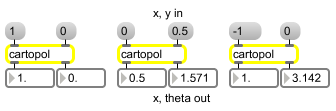cartopol

Cartesian to Polar coordinate conversion

Description

cartopol converts a pair of cartesian coordinates into a pair of polar coordinates.

None.

Messages

 bang A bang will output the most recently stored coordinate pair conversion. int coordinate [int] Converted to float. float coordinate [float] In left inlet: The x coordinate of a Cartesian pair to be converted into a polar coordinate pair consisting of distance and angle values. When used in an audio context, the value represents the real part of a frequency domain value to be converted into a polar coordinate pair consisting of amplitude and phase values. In right inlet: The y coordinate of a Cartesian pair to be converted into a polar coordinate pair consisting of distance and angle values. When used in an audio context, the value represents the imaginary part of a frequency domain value to be converted into a polar coordinate pair consisting of amplitude and phase values.

Output

float: Out left outlet: The distance portion of the polar coordinate pair. When used in an audio context, the value represents the magnitude (amplitude) of the frequency represented by the currently input.

Out right outlet: The angle portion of the polar coordinate pair. When used in an audio context, the value represents the phase, expressed in radians, of the frequency represented by the current input. If only the left outlet is connected, the phase computation is not performed.

ExamplesConvert Cartesian to Polar coordinates